# 10 Fitness Misconceptions You Need to Stop Believing

Health
9 months ago

In the modern world, sports are almost as popular as they used to be in ancient times. Every other person goes to the gym, does yoga at home, or runs in the morning. The number of myths regarding fitness has inevitably increased along with the number of people who are trying to keep fit.Here is the proof.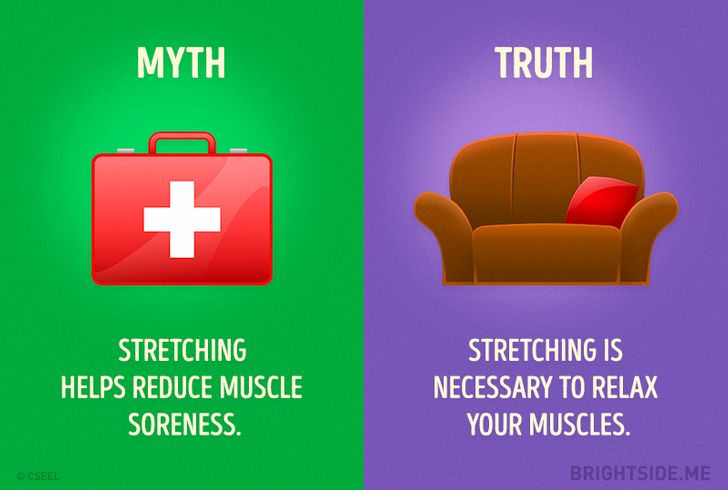Here is the proof.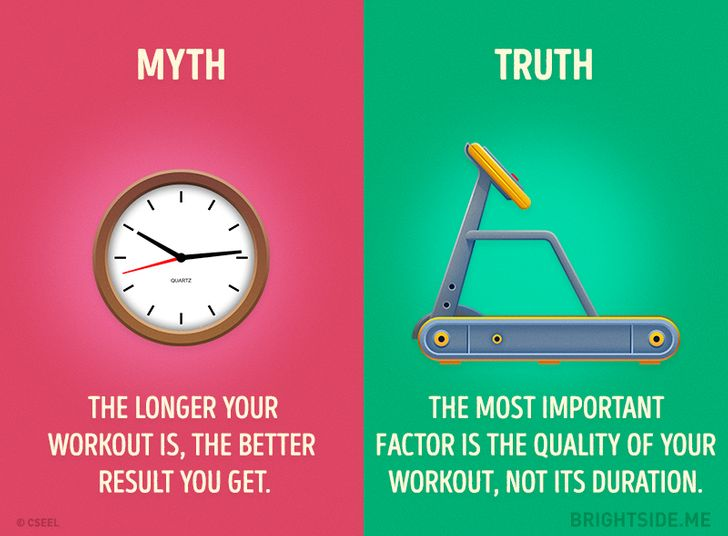Here is the proof.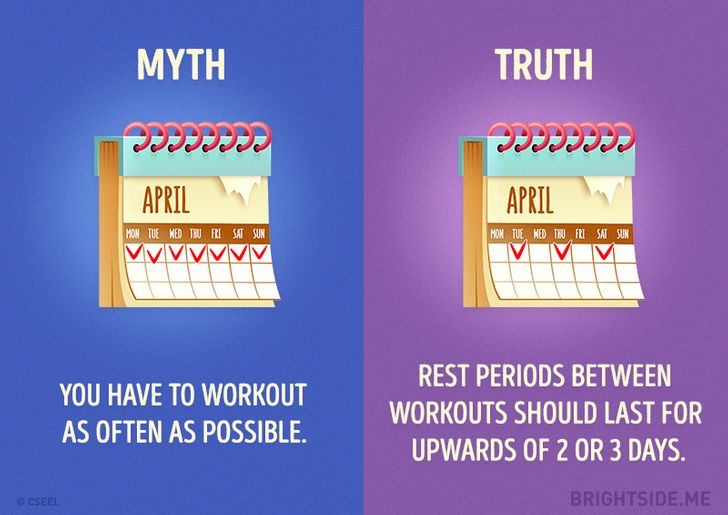Here is the proof.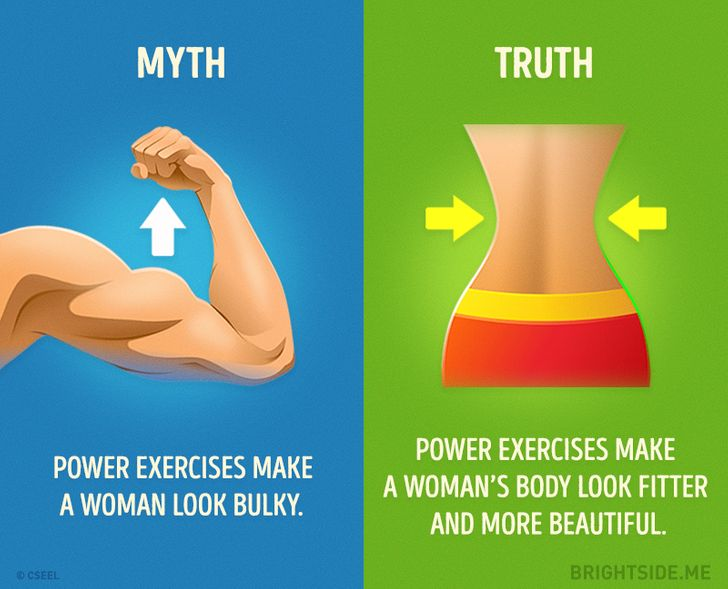Here is the proof.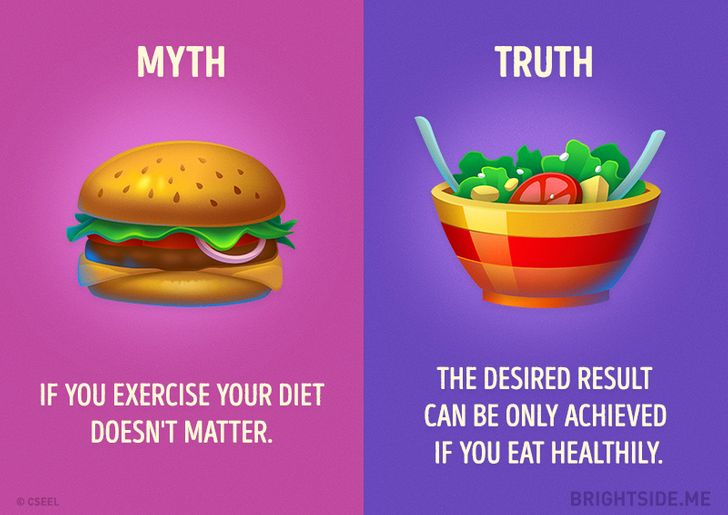Here is the proof.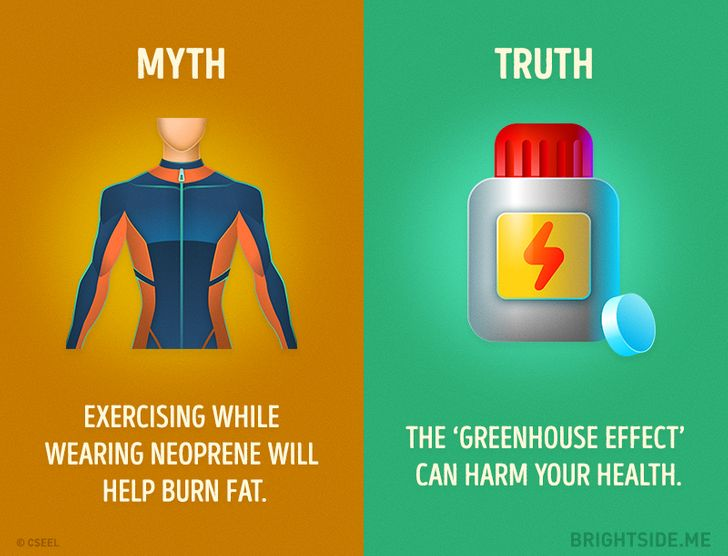Here is the proof.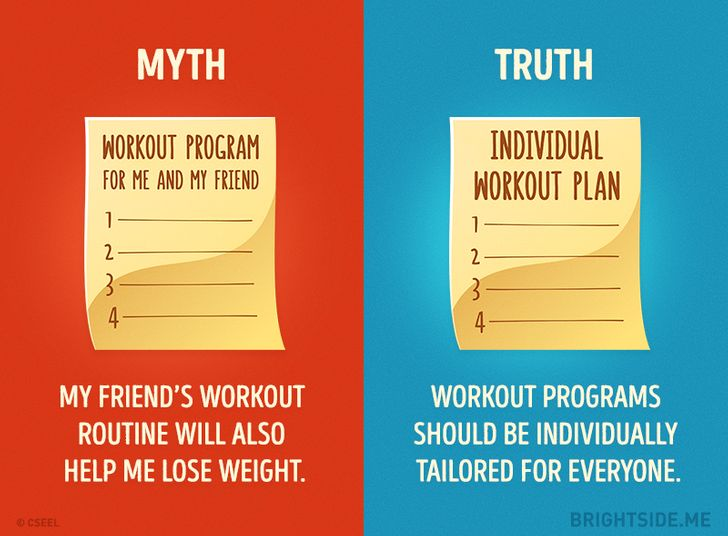Here is the proof.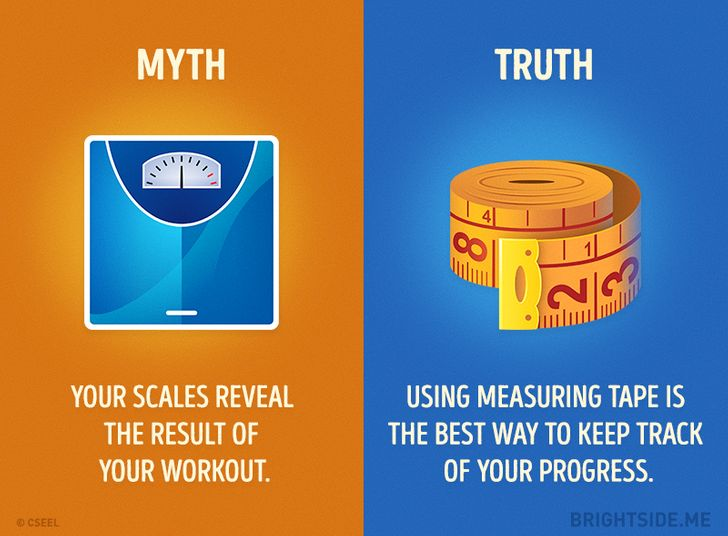Here is the proof.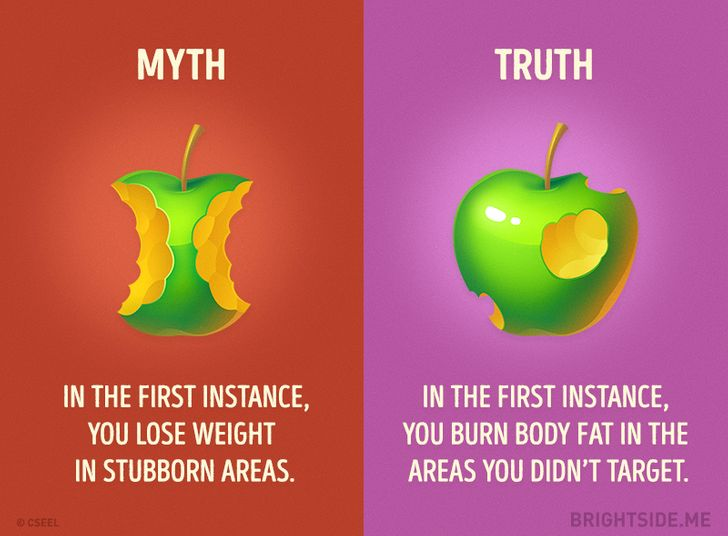Here is the proof.

Do you exercise? If so, how often? Do you do it because you want to lose weight or because it makes you feel energized?